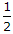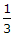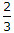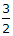# Civil Engineering - Theory of Structures

Exercise : Theory of Structures - Section 2
11.
The strain energy stored in a spring when subjected to greatest load without being permanently distorted, is called
stiffness
proof resilience
proof stress
Explanation:
No answer description is available. Let's discuss.

12.
If a three hinged parabolic arch, (span l, rise h) is carrying a uniformly distributed load w/unit length over the entire span,
horizontal thrust is wl2/8h
S.F. will be zero throughout
B.M. will be zero throughout
all the above.
Explanation:
No answer description is available. Let's discuss.

13.
Co-efficient of wind resistance of a circular surface, isExplanation:
No answer description is available. Let's discuss.

14.
Pick up the correct statement from the following:
For a uniformly distributed load, the shear force varies linearly
For a uniformly distributed load, B.M. curve is a parabola
For a load varying linearly, the shear force curve is a parabola
For a load varying linearly, the B.M. curve is a cubic parabola
All the above.